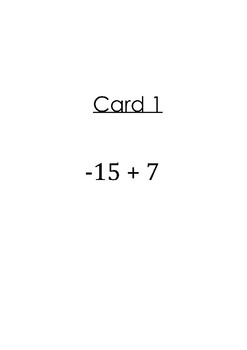# Scoot Operations with Integers!Subject
Resource Type
File Type

PDF

(2 MB)
Product Rating
Standards
• Product Description
• StandardsNEW

Awesome activity to review of operations with integers. This activity gets students up and moving around the classroom.

Solve real-world and mathematical problems involving the four operations with rational numbers.
Apply properties of operations as strategies to multiply and divide rational numbers.
Understand that integers can be divided, provided that the divisor is not zero, and every quotient of integers (with non-zero divisor) is a rational number. If 𝘱 and 𝘲 are integers, then –(𝘱/𝘲) = (–𝘱)/𝘲 = 𝘱/(–𝘲). Interpret quotients of rational numbers by describing real-world contexts.
Understand that multiplication is extended from fractions to rational numbers by requiring that operations continue to satisfy the properties of operations, particularly the distributive property, leading to products such as (–1)(–1) = 1 and the rules for multiplying signed numbers. Interpret products of rational numbers by describing real-world contexts.
Apply and extend previous understandings of multiplication and division and of fractions to multiply and divide rational numbers.
Total Pages
N/A
N/A
Teaching Duration
45 minutes
Report this Resource to TpT
Reported resources will be reviewed by our team. Report this resource to let us know if this resource violates TpT’s content guidelines.